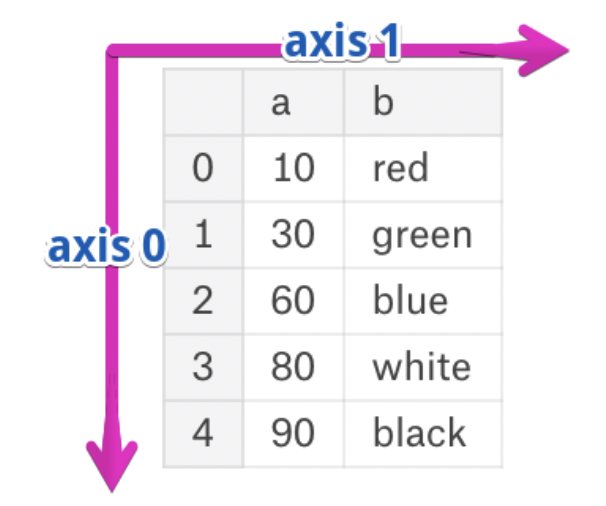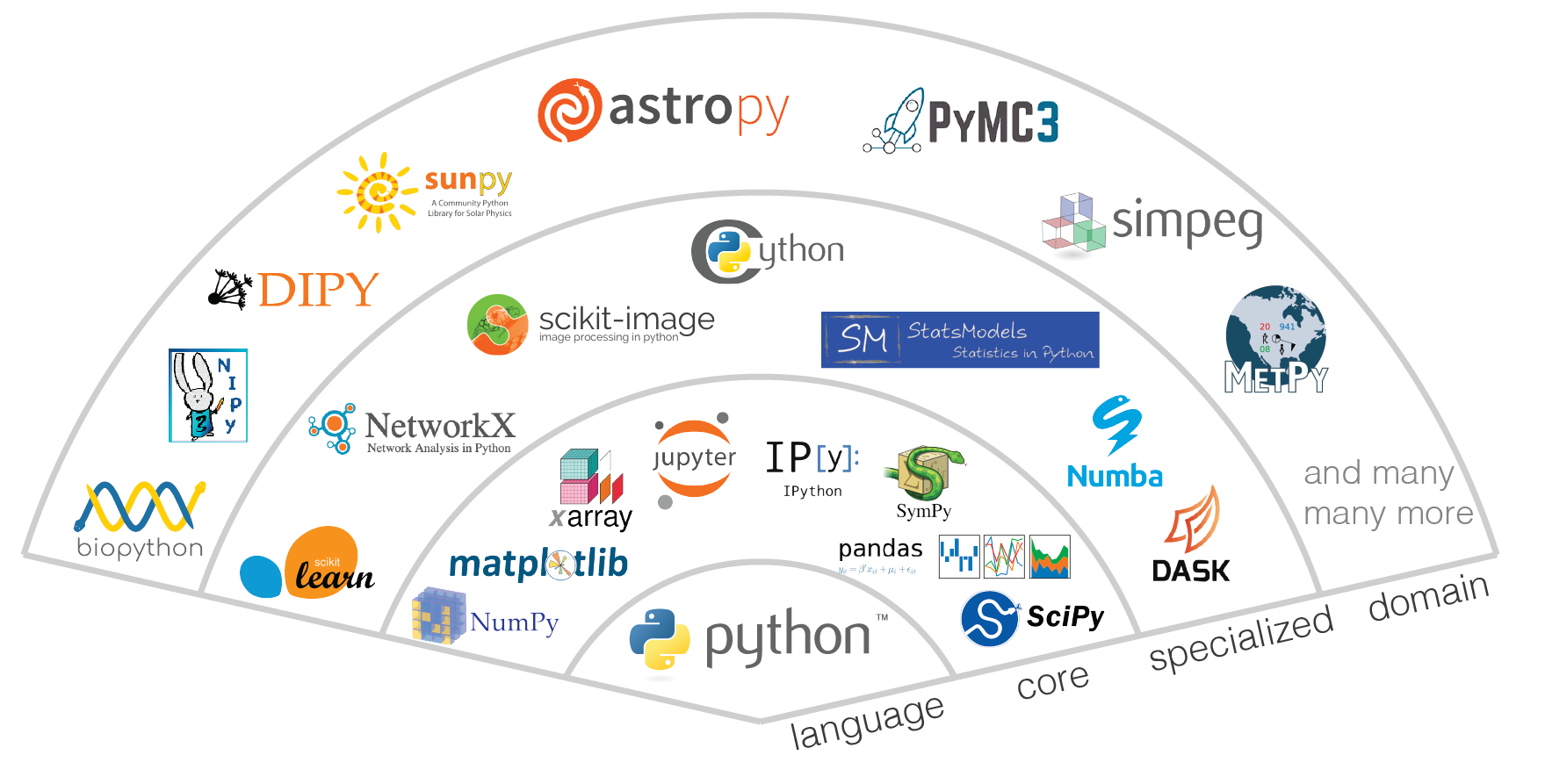# Lecture 3 – More DataFrame Fundamentals¶

## DSC 80, Spring 2023¶

### Agenda¶

• Recap: loc and iloc.
• Axes.
• pandas and numpy.
• Extra: Data cleaning and plotly.

## Recap: loc and iloc¶

### Example: Universities in California 📚¶

Recall, last lecture we started working with a dataset that contains the name, location, enrollment, and founding date of most UCs and CSUs.

### loc and iloc with the default index¶

• We use loc to access rows by their indexes (labels).
• We use iloc to access rows by their integer positions.
• When we load a DataFrame from file, the default index is 0, 1, 2, 3, ...
• In some cases, loc and iloc behave similarly – but they are not the same!

What's the difference between the two DataFrames below?

Which of the following two expressions evaluate to the name of the youngest school in schools?

### Adding and modifying columns, using a copy¶

• To add a new column to a DataFrame, use the assign method.
• To change the values in a column, add a new column with the same name as the existing column.
• Like most pandas methods, assign returns a new DataFrame.
• Pro ✅: This doesn't inadvertently change any existing variables.
• Con ❌: It is not very space efficient, as it creates a new copy each time it is called.

As an aside, you should try your best to write chained pandas code, as follows:

You can also use assign when the desired column name has spaces, by using keyword arguments.

### Adding and modifying columns, in-place¶

• You can assign a new column to a DataFrame in-place using [].
• This works like dictionary assignment.
• This modifies the underlying DataFrame, unlike assign, which returns a new DataFrame.
• This is the more "common" way of adding/modifying columns.
• ⚠️ Warning: Exercise caution when using this approach, since this approach changes the values of existing variables.

Note that we never reassigned schools_copy in the two cells above – that is, we never wrote schools_copy = ... – though it was still modified.

### Mutability¶

DataFrames, like lists, arrays, and dictionaries, are mutable. As you learned in DSC 20, this means that they can be modified after being created.

Not only does this explain the behavior on the previous slide, but it also explains the following:

Note that schools was modified, even though we didn't reassign it! These unintended consequences can influence the behavior of test cases on labs and projects, among other things!

To avoid this, it's a good idea to include df = df.copy() as the first line in functions that take DataFrames as input.

You can add and modify rows using loc and iloc. There's a function that can be to add rows, called pd.concat; we'll see it in a few lectures.

## Axes¶

### Axes¶

• The rows and columns of a DataFrame are both stored as Series.
• The axis specifies the direction of a "slice" of a DataFrame.• Axis 0 refers to the index (rows).
• Axis 1 refers to the columns.

### DataFrame methods with axis¶

Consider the DataFrame A defined below using a dictionary.

If we specify axis=0, A.sum will "compress" along axis 0, and keep the column labels intact.

If we specify axis=1, A.sum will "compress" along axis 1, and keep the row labels (index) intact.What's the default axis?

### DataFrame methods with axis¶

• In addition to sum, many other Series methods work on DataFrames.
• In such cases, the DataFrame method usually applies the Series method to every row or column.
• Many of these methods accept an axis argument; the default is usually axis=0.

### Discussion Question¶

In words, what characteristic do all schools in the following DataFrame share?

schools[schools.nunique(axis=1) != schools.nunique(axis=1).max()]


Hint: What city is SDSU in? What county is it in?

## pandas and numpy¶### numpy¶

• NumPy stands for "numerical Python". It is a commonly-used Python module that enables fast computation involving arrays and matrices.
• numpy's main object is the array. In numpy, arrays are:
• Homogenous – all values are of the same type.
• (Potentially) multi-dimensional.
• Computation in numpy is fast because:
• Much of it is implemented in C.
• numpy arrays are stored more efficiently in memory than, say, Python lists.
• This site provides a good overview of numpy arrays.

### pandas is built upon numpy¶

• A Series in pandas is a numpy array with an index.
• A DataFrame is like a dictionary of columns, each of which is a numpy array.
• Many operations in pandas are fast because they use numpy's implementations.
• To access the array underlying a DataFrame or Series, use the to_numpy method.
• ⚠️ Warning: to_numpy returns a view of the original object, not a copy! Read more in the course notes.
• .values is a soon-to-be-deprecated version of .to_numpy().

Even though conv appears to be "detached" from ser, it is not:

### The dangers of for-loops¶

• for-loops are slow when processing large datasets. You will rarely write for-loops in DSC 80, and may be penalized on assignments for using them when unnecessary!
• One of the biggest benefits of numpy is that it supports vectorized operations.
• If a and b are two arrays of the same length, then a + b is a new array of the same length containing the element-wise sum of a and b.
• To illustrate how much faster numpy arithmetic is than using a for-loop, let's compute the distances between the origin $(0, 0)$ and 1000 random points $(x, y)$ in $\mathbb{R}^2$:
• Using a for-loop.
• Using vectorized arithmetic, through numpy.

### Aside: Generating data¶

• First, we need to create a DataFrame containing 1000 random points in 2D.
• np.random.random(N) returns an array containing N numbers selected uniformly at random from the interval $[0, 1)$.

Next, let's define a function that takes in a DataFrame like coordinates and returns the distances between each point and the origin, using a for-loop.

The %timeit magic command can repeatedly run any snippet of code and give us its average runtime.

Now, using a vectorized approach:

Note that "µs" refers to microseconds, which are one-millionth of a second, whereas "ms" refers to milliseconds, which are one-thousandth of a second.

Takeaway: Avoid for-loops whenever possible!

### pandas data types¶

• Each Series (column) has a data type, which refers to the type of the values stored within. Access it using the dtypes attribute.
• A column's data type determines which operations can be applied to it.
• pandas tries to guess the correct data types for a given DataFrame, and is often wrong.
• This can lead to incorrect calculations and poor memory/time performance.
• As a result, you will often need to explicitly convert between data types.

### pandas data types¶

Pandas dtype Python type NumPy type SQL type Usage
int64 int int_, int8,...,int64, uint8,...,uint64 INT, BIGINT Integer numbers
float64 float float_, float16, float32, float64 FLOAT Floating point numbers
bool bool bool_ BOOL True/False values
datetime64 NA datetime64[ns] DATETIME Date and time values
timedelta[ns] NA NA NA Differences between two datetimes
category NA NA ENUM Finite list of text values
object str string, unicode NA Text
object NA object NA Mixed types

This article details how pandas stores different data types under the hood.

What do you think is happening here? 🚰

Read this article for a discussion of how numpy/pandas int64 operations differ from vanilla int operations.

### ⚠️ Warning: numpy and pandas don't always make the same decisions!¶

numpy prefers homogenous data types to optimize memory and read/write speed. This leads to type coercion.

Notice that the array created below contains only strings, even though there was an int in the argument list.

On the other hand, pandas likes correctness and ease-of-use. The Series created below is of type object, which preserves the original data types in the argument list.

You can specify the data type of an array when initializing it by using the dtype argument.

pandas does make some trade-offs for efficiency, however. For instance, a Series consisting of both ints and floats is coerced to the float64 data type.

### Type conversion¶

You can change the data type of a Series using the .astype Series method.

For instance, we can change the data type of the 'Enrollment' column in schools to be int64, once we remove the commas.

### Performance and memory management¶

As we just discovered,

• numpy is optimized for speed and memory consumption.
• pandas makes implementation choices that:
• are slow and use a lot of memory, but
• optimize for fast code development.

To demonstrate, let's create a large array in which all of the entries are non-negative numbers less than 255, meaning that they can be represented with 8 bits (i.e. as np.uint8s, where the "u" stands for "unsigned").

When we tell pandas to use a dtype of uint8, the size of the resulting DataFrame is under a megabyte.

But by default, even though the numbers are only 8-bit, pandas uses the int64 dtype, and the resulting DataFrame is over 7 megabytes large.

### Aside: std¶

To compute the standard deviation of a Series, we can use:

• The std method.
• The np.std function.

Let's try both. What do you notice?

### Aside: std¶

The two methods/functions use different degrees of freedom (ddof) by default.

• The std method in pandas uses ddof=1 by default (sometimes called the "sample" standard deviation):
$$\text{SD} = \sqrt{\frac{\sum_{i = 1}^n (x_i - \bar{x})^2}{n - 1}}$$
• The np.std method in numpy uses ddof=0 by default (sometimes called the "population" standard deviation):
$$\text{SD} = \sqrt{\frac{\sum_{i = 1}^n (x_i - \bar{x})^2}{n}}$$

Be careful!

## Extra: Data cleaning and plotly¶

Note: We may not get to these slides in lecture; refer to them for extra examples.

### Example: Universities in California 📚¶

Let's return to schools. Towards the end of the last section, we fixed the data type of the 'Enrollment' column to be int64, which means we can now perform calculations with it.

### plotly¶

plotly is a plotting library that creates interactive graphs. It's not included in your dsc80 conda environment, so you'll need to pip install it.